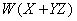Computer Organization and Structure

Homework #1

Due: 2003/10/14

1.      Which of the following contain circuits that are likely to be combinational and which contain sequential circuits? Explain your rationale.

a.         A washing machine that sequences through the soak, wash, and spin cycles for preset periods of time.

b.        A three-input majority circuit that outputs a logic 1 if any two of its inputs are 1.

c.         A circuit that divides two 2-bit numbers to yield a quotient and a remainder.

d.        A machine that takes a dollar bill and gives three quarters, two dimes, and a nickel in change, one at a time through a single coin change slot.

e.         A digital alarm clock that generates an alarm when a preset time has been reached.

2.      Write truth tables for the following three functions.

a.         A 2-bit-wide shifter takes two input signals,and, and shifts them to two outputs,and, under the control of a shift signal. If this signal SHIFT is false, then the inputs are connected straight through to the outputs. If SHIFT is true, thenis routed toandshould be set to a.

b.        A 1-bit demultiplexer takes an input signal IN and shifts it to one of two outputs,and, under the control of a single SELECT signal. If SELECT is, then IN is connected through toandis connected to a. If SELECT is, then IN is connected through toandis connected to a.

c.         A 2-bit multiplexer takes two input signals,and, and shifts one of them to the single output OUT under the control of a 1-bit select signal. If the SELECT signal is false, thenis passed to OUT. If SELECT is true, thenis passed to OUT.

3.      Write sum of products expressions for the above truth tables.

4.      Given the above Boolean expressions, draw logic schematics using AND, OR, and INVERT gates that implement those functions.

5.      Draw schematics for the following functions in terms of AND, OR, and INVERT gates.

a.b.c.d.e.6.      Prove the following simplification theorems using the first eight laws of Boolean algebra:

a.b.c.d.7.      Prove, using truth tables, that.

8.      Simplify the following functions using the theorems of Boolean algebra. Write the particular law or theorem you are using in each step. For each simplified function you derive, how many literals does it have?

a.b.c.d.e.9.      Using K-maps, find the minimum sum of products form for the functionand its complement.

10.  Consider a five-input Boolean function that is asserted whenever exactly two of its inputs are asserted.

a.         Construct its truth table.

b.        What is the function in sum of products form, using “little m” notation?

c.         What is the function in product of sums form, using “big M” notation?

d.        Use the Karnaugh map method to simplify the function in sum of products form.